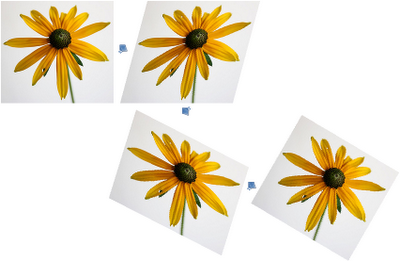### Rotate image in Paint

It is hard to impress people with computer drawing of a car nowadays. However, if you do it with MS Paint, the impression could be completely different. But even the most prominent mspaint wizards will probably say that Paint can't rotate images by arbitrary angle: only rotations by 90°, 180° and 270° are supported. Rotating a picture by, for example, 30° is simply not possible, is it?

Well, with mathematics, a workaround can be found:Rotating image (original) by 30° with 3 shears, useless but curious.

The key is the shear transform, which is supported by some reason (I guess, because it is simple to implement). If you shear a picture once, it will get distorted, not rotated. It may seem that shearing twice can solve the problem, but alas, two shears are also not enough. However, 3 finely tuned shears can produce pure rotation, with all distortions cancelled out. Here is some math.

Mathematically, both rotation and shears are linear transformations, described by the square rank 2 matrices:

$$R(\phi) = \begin{bmatrix}\cos\phi & -\sin\phi \\ \sin\phi & \cos\phi \end{bmatrix},$$ $$S_H(\phi) = \begin{bmatrix}1&\tan\phi\\0&1\end{bmatrix}, S_V(\phi) = \begin{bmatrix}1&0\\\tan\phi&1\end{bmatrix}.$$

Here, R is the rotation matrix, SH is the horizontal shear matrix, and SV is the vertical shear matrix, and φ is the angle of rotation or shear. Since combining transforms is equivalent to multiplying their matrices, the problem can be reformulated as: decompose rotation matrix R into matrix product of several shear matrices.

Like this:

$$R(\phi) = S_H(\phi/2)\cdot S_V(-\arctan\sin\phi)\cdot S_H(\phi/2),$$ because it can be easily checked that: $$\begin{bmatrix}\cos\phi & -\sin\phi \\ \sin\phi & \cos\phi \end{bmatrix} = \begin{bmatrix}1&\tan(\phi/2)\\0&1\end{bmatrix}\cdot \begin{bmatrix}1&0\\-\sin\phi&1\end{bmatrix}\cdot \begin{bmatrix}1&\tan(\phi/2)\\0&1\end{bmatrix}.$$

In other words, translating from math to English: to rotate by φ, shear horizontally by φ/2, then vertically by -arctan(sin(φ)), then again horizontally by φ/2.

### Calculator for shear angles

Enter desired rotation angle and shear 3 times according to instruction. For the best result, angle should be in the interval [-45°; 45°]. Allowed interval is [-178°; 178°], but big shear angle can produce extremely large images. Since Paint can only shear by integer angle, calculator automatically rounds results. (You need to enable Java script to use it).

To rotate by °:

## Further improvements

### Better angle approximation

Paint only shear shears by integer angle, if measured in degrees. Values, given by the formula above, are generally non-integer and must be rounded. This causes additional distortion, result is slightly different from exact rotation.

The solution is to replace 1 shear with 2 or more consecutive shears in the same direction. The law for combining shears is: $$\tan\alpha+\tan\beta=\tan\gamma,$$ where $$\alpha,\beta,\gamma$$ are shear angles. Thus to shear by 26.565°, instead of shearing by 27° (relative error is 1.6%) one may shear by 11° and then by 17°. This will give total shear angle of 26.570°, with relative error 0.018%. That's some 87 times better! Improved algorithm for rotation by 30° is:

• Shear horizontally by 15°
• Vertically by -11°.
• Vertically by -17°.
• Again horizontally by 15°.
Using 3 and more shears can give even better approximation, however, each shear introduces distortions, caused by rounding and using to many of them may diminish effect of better approximation.

Another example: to rotate by 45°, use the following sequence of 6 shears: H 4°; H 19°; V 10°; V 28°; H 4°; H 19°. This gives overall transform very close to the true rotation, but image will be noisy because of big numbers of transforms.

### Image interpolation

When shearing image, Paint uses nearest-point approximation, moving each original pixel by integer amount. This causes rotated images to become "noisy", each pixel shifted by some random value. To overcome this, stretch image before rotation (400% is just fine), then shrink it back after. Shrinking will make image smoother.